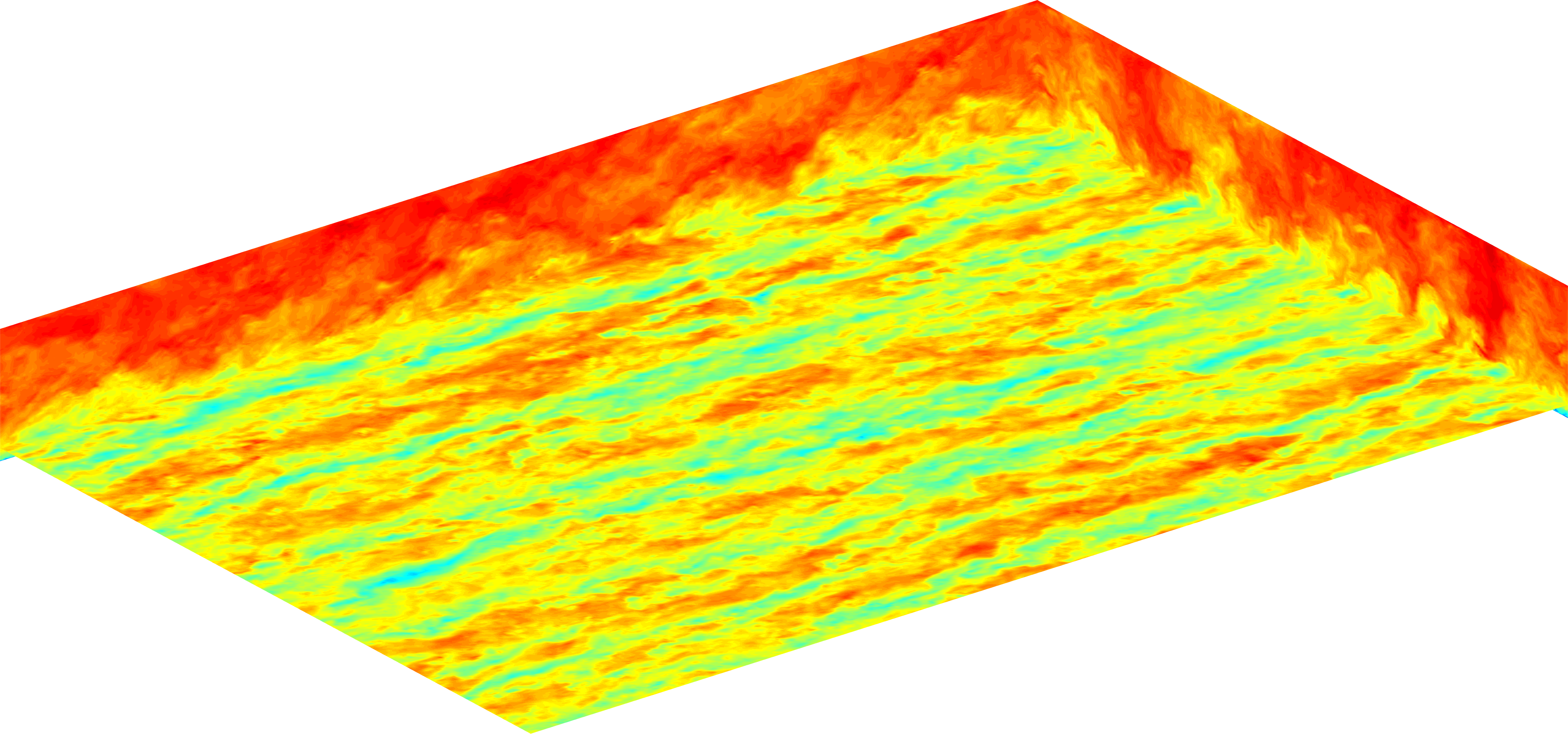# LESGOLESGO is a parallel pseudo-spectral large-eddy simulation code.

# Level set immersed boundary method

For representing solid objects in the flow domain, the level set immersed boundary method is used. All objects are represented using a level set or signed distance function (phi) where phi = 0 on the surface, phi < 0 inside objects and phi > 0 otherwise. For each grid point in the computational domain the minimum distance to any one of the objects in the domain must be computed and assigned to the variable phi using a preprocessing program. The code will then use phi to compute the surface normals which is then used to apply the log-law stress within a small “band” along a the surface boundary. For all grid points on or inside of the surface the velocity is forced to zero using a direct forcing approach.

## Usage

To use the level set modules in a simulation, a file “phi.out” containing the signed distance function data must be created first. This must be generated with a separate program or using the built-in “trees_pre_ls” functionality. It is recommended making the signed distance function exact if possible, or at least sampled at a higher resolution than the computational grid. There are many adjustable parameters within the level set module that control how the boundary conditions at the level set surface are applied. These are listed in the input file lesgo.conf within the LEVEL_SET block. If you encounter problems (e.g., kinks in velocity profiles), try adjusting some of these (e.g., length scale parameters that control how close a given point must be to the surface before an certain action is taken). The variables “nphitop”, “nphibot”, etc, control how many extra z-levels are copied between MPI processes when determining boundary conditions (at top and bottom of the process-local domain).

## Creating trees with trees_pre_ls

The built-in “trees_pre_ls” functionality may be used for generating the “phi” field for the level set module. This generates fractal trees with either round or square branches. In addition to trees, it may also be used to easily generate an array of wall mounted cubes which is commonly used as one of the test cases for lesgo.

To use this feature, simply enable the use_trees the LVLSET block and write a “trees.conf” file. The file “trees.conf” is the input file for trees_pre_ls (“lesgo.conf” is also required by trees_pre_ls). An example “trees.conf” is:

# This is a trees configuration file
# this is a comment, it begins with a #

n_tree = 1

# see trees_setup_ls.f90 to see what each of these parameters does
tree = {
n_gen = 2
n_sub_branch = 4
l = 0.3125
d = 0.125
#x0 = 0.5, 0.5, 0.0078125
x0 = 0.5, 0.5, 0.0
taper = 0.
ratio = 0.48
rel_dir = -0.4924038763,-0.8528685321,-0.1736481773,
0.0000000000,0.0000000000,1.0000000000,
-0.4924038763,0.8528685320,-0.1736481773,
0.9848077530,0.0000000000,-0.1736481773
root_height = 0.75, 1.0, 0.75, 0.75
twist = -90.0, 0.0, -90.0, -90.0
#trunk_twist = 90.0
max_res_gen=1
}


This creates a tree structure with 2 generations of branches (the trunk counts as generation zero), with each branch having 4 sub-branches. The length of each the trunk is 0.3125 and the diameter of the trunk is 0.125. If the add_cap option is true, then the actual length of the tree trunk will be $$l + \frac{d}{2} = 0.3125 + \frac{0.125}{2} = 0.375$$. The center of the base of the trunk is at $$x_0$$ (given as x, y, z coordinates). The branches are not tapered. The ratio of lengths between a branch and each of its sub-branches is 0.48. The directions of the sub-branches, relative to the parent-branch coordinate system are sub-branch 1: (-0.49, -0.85, -.17) sub-branch 2: (0, 0, 1) sub-branch 3: (-0.49, 0.85, -0.17) sub-branch 4: (0.98, 0, -0.17) Three sub-branches are placed 75 % of the way along the parent branch (root_height), and one is at the top of the parent branch. Each sub-branch has a twist about its own branch axis applied to it. The trunk can be twisted separately, but this line has been commented out.
Even if you want to simulate more that one unresolved branch generation, right now the code expects max_res_gen + 1 = n_gen. Note that although the relations between a branch and its sub-branches are defined in trees.conf, iterated function systems (IFS) are not used to describe the trees. Instead, a sub-branch is defined only by reference to its parent branch.

The level set function required by the level set routines will be calculated at before the first timestep of each lesgo run.